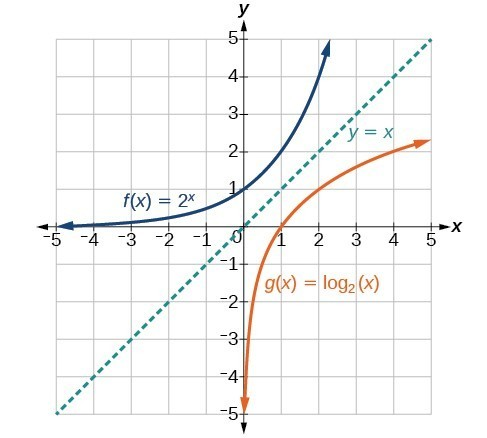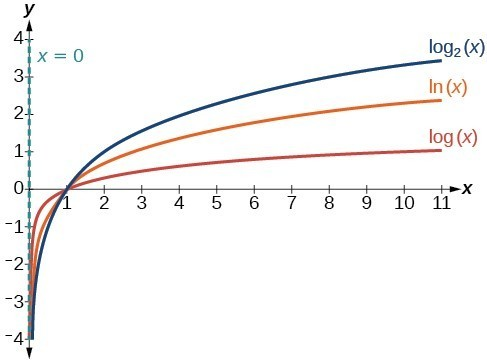## Characteristics of Graphs of Logarithmic Functions

### Learning Outcomes

• Determine the domain and range of a logarithmic function.
• Determine the x-intercept and vertical asymptote of a logarithmic function.
• Identify whether a logarithmic function is increasing or decreasing and give the interval.
• Identify the features of a logarithmic function that make it an inverse of an exponential function.

Before working with graphs, we will take a look at the domain (the set of input values) for which the logarithmic function is defined.

Recall that the exponential function is defined as $y={b}^{x}$ for any real number x and constant $b>0$, $b\ne 1$, where

• The domain of y is $\left(-\infty ,\infty \right)$.
• The range of y is $\left(0,\infty \right)$.

In the last section we learned that the logarithmic function $y={\mathrm{log}}_{b}\left(x\right)$ is the inverse of the exponential function $y={b}^{x}$. So, as inverse functions:

• The domain of $y={\mathrm{log}}_{b}\left(x\right)$ is the range of $y={b}^{x}$: $\left(0,\infty \right)$.
• The range of $y={\mathrm{log}}_{b}\left(x\right)$ is the domain of $y={b}^{x}$: $\left(-\infty ,\infty \right)$.

Transformations of the parent function $y={\mathrm{log}}_{b}\left(x\right)$ behave similarly to those of other functions. Just as with other parent functions, we can apply the four types of transformations—shifts, stretches, compressions, and reflections—to the parent function without loss of shape.

Previously we saw that certain transformations can change the range of $y={b}^{x}$. Similarly, applying transformations to the parent function $y={\mathrm{log}}_{b}\left(x\right)$ can change the domain. Therefore, when finding the domain of a logarithmic function, it is important to remember that the domain consists only of positive real numbers. That is, the value you are applying the logarithmic function to, also known as the argument of the logarithmic function, must be greater than zero.

For example, consider $f\left(x\right)={\mathrm{log}}_{4}\left(2x - 3\right)$. This function is defined for any values of x such that the argument, in this case $2x - 3$, is greater than zero. To find the domain, we set up an inequality and solve for x:

$\begin{array}{l}2x - 3>0\hfill & \text{Show the argument greater than zero}.\hfill \\ 2x>3\hfill & \text{Add 3}.\hfill \\ x>1.5\hfill & \text{Divide by 2}.\hfill \end{array}$

In interval notation, the domain of $f\left(x\right)={\mathrm{log}}_{4}\left(2x - 3\right)$ is $\left(1.5,\infty \right)$.

### How To: Given a logarithmic function, identify the domain

1. Set up an inequality showing the argument greater than zero.
2. Solve for x.
3. Write the domain in interval notation.

### Example: Identifying the Domain Resulting From a Logarithmic Shift

What is the domain of $f\left(x\right)={\mathrm{log}}_{2}\left(x+3\right)$?

### Try It

What is the domain of $f\left(x\right)={\mathrm{log}}_{5}\left(x - 2\right)+1$?

### Example: Identifying the Domain Resulting From a Logarithmic Shift and Reflection

What is the domain of $f\left(x\right)=\mathrm{log}\left(5 - 2x\right)$?

### Try It

What is the domain of $f\left(x\right)=\mathrm{log}\left(x - 5\right)+2$?

## Graphing a Logarithmic Function Using a Table of Values

Now that we have a feel for the set of values for which a logarithmic function is defined, we move on to graphing logarithmic functions. The family of logarithmic functions includes the parent function $y={\mathrm{log}}_{b}\left(x\right)$ along with all of its transformations: shifts, stretches, compressions, and reflections.

We begin with the parent function $y={\mathrm{log}}_{b}\left(x\right)$. Because every logarithmic function of this form is the inverse of an exponential function of the form $y={b}^{x}$, their graphs will be reflections of each other across the line $y=x$. To illustrate this, we can observe the relationship between the input and output values of $y={2}^{x}$ and its equivalent logarithmic form $x={\mathrm{log}}_{2}\left(y\right)$ in the table below.

 x –3 –2 –1 0 1 2 3 ${2}^{x}=y$ $\frac{1}{8}$ $\frac{1}{4}$ $\frac{1}{2}$ 1 2 4 8 ${\mathrm{log}}_{2}\left(y\right)=x$ –3 –2 –1 0 1 2 3

Using the inputs and outputs from the table above, we can build another table to observe the relationship between points on the graphs of the inverse functions $f\left(x\right)={2}^{x}$ and $g\left(x\right)={\mathrm{log}}_{2}\left(x\right)$.

 $f\left(x\right)={2}^{x}$ $\left(-3,\frac{1}{8}\right)$ $\left(-2,\frac{1}{4}\right)$ $\left(-1,\frac{1}{2}\right)$ $\left(0,1\right)$ $\left(1,2\right)$ $\left(2,4\right)$ $\left(3,8\right)$ $g\left(x\right)={\mathrm{log}}_{2}\left(x\right)$ $\left(\frac{1}{8},-3\right)$ $\left(\frac{1}{4},-2\right)$ $\left(\frac{1}{2},-1\right)$ $\left(1,0\right)$ $\left(2,1\right)$ $\left(4,2\right)$ $\left(8,3\right)$

As we would expect, the and y-coordinates are reversed for the inverse functions. The figure below shows the graphs of f and g.Notice that the graphs of $f\left(x\right)={2}^{x}$ and $g\left(x\right)={\mathrm{log}}_{2}\left(x\right)$ are reflections about the line y = x since they are inverses of each other.

Observe the following from the graph:

• $f\left(x\right)={2}^{x}$ has a y-intercept at $\left(0,1\right)$ and $g\left(x\right)={\mathrm{log}}_{2}\left(x\right)$ has an x-intercept at $\left(1,0\right)$.
• The domain of $f\left(x\right)={2}^{x}$, $\left(-\infty ,\infty \right)$, is the same as the range of $g\left(x\right)={\mathrm{log}}_{2}\left(x\right)$.
• The range of $f\left(x\right)={2}^{x}$, $\left(0,\infty \right)$, is the same as the domain of $g\left(x\right)={\mathrm{log}}_{2}\left(x\right)$.

### A General Note: Characteristics of the Graph of the Parent Function $f\left(x\right)={\mathrm{log}}_{b}\left(x\right)$

For any real number x and constant > 0, $b\ne 1$, we can see the following characteristics in the graph of $f\left(x\right)={\mathrm{log}}_{b}\left(x\right)$:

• one-to-one function
• vertical asymptote: = 0
• domain: $\left(0,\infty \right)$
• range: $\left(-\infty ,\infty \right)$
• x-intercept: $\left(1,0\right)$ and key point $\left(b,1\right)$
• y-intercept: none
• increasing if $b>1$
• decreasing if 0 < < 1

The graphs below show how changing the base b in $f\left(x\right)={\mathrm{log}}_{b}\left(x\right)$ can affect the graphs. Observe that the graphs compress vertically as the value of the base increases. (Note: recall that the function $\mathrm{ln}\left(x\right)$ has base $e\approx 2.718$The graphs of three logarithmic functions with different bases, all greater than 1.

### How To: Given a logarithmic function Of the form $f\left(x\right)={\mathrm{log}}_{b}\left(x\right)$, graph the function

1. Draw and label the vertical asymptote, x = 0.
2. Plot the x-intercept, $\left(1,0\right)$.
3. Plot the key point $\left(b,1\right)$.
4. Draw a smooth curve through the points.
5. State the domain, $\left(0,\infty \right)$, the range, $\left(-\infty ,\infty \right)$, and the vertical asymptote, x = 0.

### Example: Graphing a Logarithmic Function Of the Form $f\left(x\right)={\mathrm{log}}_{b}\left(x\right)$

Graph $f\left(x\right)={\mathrm{log}}_{5}\left(x\right)$. State the domain, range, and asymptote.

### Try It

Graph $f\left(x\right)={\mathrm{log}}_{\frac{1}{5}}\left(x\right)$. State the domain, range, and asymptote.

## Contribute!

Did you have an idea for improving this content? We’d love your input.